Sponsored Link •Weblogs Forum
Metaclasses in Python 3.0 [2 of 2]

0 replies on 1 page.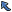Back to Topic List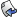Reply to this Topic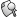Search Forum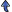Threaded View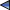Previous Topic Next Topic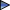Flat View: This topic has 0 replies on 1 page
 Michele Simionato Posts: 222 Nickname: micheles Registered: Jun, 2008
Metaclasses in Python 3.0 [2 of 2] (View in Weblogs)
Posted: Aug 9, 2008 9:07 AMReply
Summary
In the first part I have discussed the new features of metaclasses in Python 3.0, in particular the usage of the __prepare__ classmethod to intercept the class attributes *before* class creation. In this second part I will show an example of the things you can do with metaclasses, by implementing a clever record system.
 Advertisement# A zoology of records

Classes are used to model sets of objects; in the same sense, metaclasses are used to model sets of classes. In order to show the power of metaclasses, we will define a set of record classes, all subclasses of a common mother class Record and all instances of the same metaclass MetaRecord, with a sum operator. In mathematical term our set of classes will be a monoid, i.e. a set with an associative composition law (the composition operator will be denoted with +) and an identity, the base class Record. Since Python tuples are already a monoid - the sum of two tuples is a tuple and the empty tuple works as an identity element - it makes sense to define our set of classes as a set of tuples. The difference between ordinary tuples and the records we will define is that records have the concept of type: every given record field has a given type. Since Python is dynamically typed the types will be specified in terms of casting functions, taking in input one or more types (for instance integers and/or strings) and returning a fixed type in output - or a TypeError if the if the input type is not acceptable. We will consider the following casting functions for records of kind Book:

```def varchar(n):
"""varchar(n) converts an object into a string with less than n
characters or raises a TypeError"""
def check(x):
s = str(x)
if len(s) > n:
raise TypeError('Entered a string longer than %d chars' % n)
return s
check.__name__ = 'varchar(%d)' % n
return check
```
```def date(x):
"Takes a string (or date) and converts it into a date"
if isinstance(x, datetime.date):
x = x.isoformat()[:10]
return datetime.date(*map(int, x.split('-')))
```
```def score(x):
"Takes a string and converts it into an integer in the range 1-5"
if set(x) != {'*'} or len(x) > 5:
raise TypeError('%r is not a valid score!' % x)
return len(x)
```

varchar(N) makes sure that the string input is shorter than N characters; for instance

```>>> varchar(128)('a'*129)
Traceback (most recent call last):
...
TypeError: Entered a string longer than 128 chars
```

date makes sure that the string in input is a data in ISO format; score converts a string with or or more stars into an integer number: the idea is that a book has a score in stars, for one to five.

```>>> score('***')
3
```
```>>> score('')
Traceback (most recent call last):
...
TypeError: '' is not a valid score!
```

We will define records like the following, where Record is a subclass of tuple enhanced with a suitable metaclass:

```class Book(Record):
title_type = varchar(128)
author_type = varchar(64)

class PubDate(Record):
date_type = date

class Score(Record):
score_type = score
```

On these records it will be possible to define a sum operator taking two or more classes and returning a new class:

```>>> Book + PubDate + Score
<class Book+PubDate+Score title:varchar(128), author:varchar(64), date:date, score:score>
```

It will be possible to verify the associativity:

```>>> (Book + PubDate) + Score == Book + (PubDate + Score)
True
```

and the existence of the identity element:

```>>> Book + Record == Book
True
```
```>>> Record + Book == Book
True
```

These properties at the class level correspond to analogous properties at the instance level. Consider for instance the null record

```>>> null = Record()
>>> null
<Record >
```

a record ot type Book

```>>> b = Book('Putting Metaclasses to Work', 'Ira Forman')
>>> b
<Book title=Putting Metaclasses to Work, author=Ira Forman>
```

and a record of type PubDate:

```>>> d = PubDate('1998-10-01')
```

You can see that null is an identity:

```>>> b + null == null + b == b
True
```

Here is an example of sum of nontrivial records:

```>>> s = b + d
>>> s
<Book+PubDate title=Putting Metaclasses to Work, author=Ira Forman, date=1998-10-01>
```

You can access the fields by name

```>>> s.title, s.author, s.date
('Putting Metaclasses to Work', 'Ira Forman', datetime.date(1998, 10, 1))
```

or by index

```>>> s, s, s
('Putting Metaclasses to Work', 'Ira Forman', datetime.date(1998, 10, 1))
```

since s is a tuple after all.

# How it works

The method __add__ of the base class Record determines the classes of the records in input, builds the class of the record in output by using MetaRecord, and instantiate it with the right values. In particolar, if the classes in input take N and M parameters respectively (in our case N=2 and M=1) the class in out will take N+M parameters (in our case title, author and publication date). Here is the code for the base class Record:

```class Record(tuple, metaclass=MetaRecord):
"Base record class, also working as identity element"

cls = type(self) + type(other)

def __repr__(self):
slots = ['%s=%s' % (n, v) for n, v in zip(self.all_names, self)]
return '<%s %s>' % (self.__class__.__name__, ', '.join(slots))
```

Since the metaclass is smart enough to keep a register of its instances, if class compatible with the class of the record in output alread exists, it is reused: in other words, we don't need to create new classes at each sum. MetaRecord defines an equivalence relation between its instances by defining the __eq__ method: two record classes are considered equivalente (compatible) if they have the same fields in the same order. Another metaclass magic is the validation of the parameters before instantiation of a concrete record, implemented by overriding by metaclass __call__ method. Here we check that we are passing the right number of parameters and we convert them into the right types or we raise an exception. Therefore the Record subclass can assume to get the right parameters and its __init__ method is simple. The record fields are accessible by name since the metaclass defines suitable properties automatically. Finally, the metaclass redefine the + operator by implementing the __add__ method in terms of subclassing, so that

```>>> BookWithScore = Book + Score
```

is the same than

```>>> class BookWithScore2(Book, Score):
...      pass
```
```>>> BookWithScore2 == BookWithScore
True
```

There is a difference, though: the sum reuses pre-existing classes if possible, whereas inheritance always creates new classes, as customary in Python. Here is the code for MetaRecord:

```class MetaRecord(type):
_repository = {} # repository of classes already instantiated

@classmethod
def __prepare__(mcl, name, bases):
return odict()

def __new__(mcl, name, bases, odic):
entered_slots = tuple((n,v) for n, v in odic.items()
if n.endswith('_type'))
all_slots = getslots(bases) + entered_slots
check_disjoint(n for (n, f) in all_slots)
# check the field names are disjoint
odic['all_slots'] = all_slots
odic['all_names'] = tuple(n[:-5] for n, f in all_slots)
odic['all_fields'] = fields = tuple(f for n, f in all_slots)
cls = super().__new__(mcl, name, bases, dict(odic))
mcl._repository[fields] = cls
for i, (n, v) in enumerate(all_slots):
setattr(cls, n[:-5], property(lambda self, i=i: self[i]))
return cls

def __eq__(cls, other):
return cls.all_fields == other.all_fields

def __ne__(cls, other):
# must be defined explicitely; overriding __eq__ only is not enough
return cls.all_fields != other.all_fields

def __call__(cls, *values):
expected = len(cls.all_slots)
passed = len(values)
if passed != expected:
raise TypeError('You passed %d parameters, expected %d' %
(passed, expected))
vals = [f(v) for (n, f), v in zip(cls.all_slots, values)]
return super().__call__(vals)

name = '%s+%s' % (cls.__name__, other.__name__)
fields = tuple(f for n, f in getslots((cls, other)))
try: # retrieve from the repository an already generated class
return cls._repository[fields]
except KeyError:
return type(cls)(name, (cls, other), {})

def __repr__(cls):
slots = ['%s:%s' % (n[:-5], f.__name__)
for (n, f) in cls.all_slots]
return '<%s %s %s>' % ('class', cls.__name__,
', '.join(slots))
```

check_disjoint and getslots are utility functions:

```def check_disjoint(names):
storage = set()
for name in names:
if name in storage:
raise NameError('Field %r occurs twice!' % name)
else:

def getslots(bases):
return sum((getattr(b, 'all_slots', ()) for b in bases), ())
```

In closing, let me notice the usage of the new super builtin: in Python 3.0 super() is actually a shortcut for super(current-class, first-argument-of-the-current-method) i.e. the Python compiler has been made smart enough to recognize the class where a method is defined and its first argument. You can also use the old syntax and actually if you are defining a method outside a class you must do so, in order to avoid ambiguities.

# Disclaimer

The code I presented here is intended for pedagogical purposes: I have no real life usage for it. If you just want tuples with named fields use the collections.namedtuple builtin, not this recipe. Generally speaking I advice not to use metaclasses, since more often than not there are simpler and less magical alternatives available. In particular the ability to use class decorators in Python 2.6/3.0 has substantially reduced the number of good use cases for metaclasses. You should never use a metaclass to introduce a feature that should not be inherited. There is an exception to this rule when you want to redefine binary operators like +, -, *, /, == or != etc at the class level, since there is no other way than using a custom metaclass in this case. However you could also consider the idea of defining suitable top-level functions (plus, minus, mul, div, eq, ne) operating on classes following some specific interface. In practice, I would say that the most common (good) use case for metaclasses is an Object Relational Mapper framework. Other than that, the good old advice of resisting to cleverness does apply.Previous Topic Next TopicWeb Artima.com